Monday 08th August 2022CBSE Guess > Papers > Important Questions > Class XI > 2010 > Maths > Maths By Mr. Anil Kumar Tondak CBSE CLASS XI
Relations and Functions Q.1. Define Modulus function.Draw its graph & mapping.What is its domain & range?; Q.2. Define Signum function.Draw its graph & mapping.What is its domain & range? Q.3. Let A = {1, 2, 3} B = {3, 4}; ; and C = {4, 5, 6} Find (A ´ B) Ç (A ´ C). Q.4. Let A =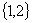B =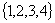C =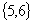D =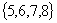Verify A´C is a subset of B´D. Q.5. If P = { 1,2 }, form the set P x P x P. Q.6. If f(x) = x3 Find;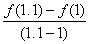Q.7. Let A = {1, 2} and B = { 3, 4}. Find the number of relations from A to B. Q.8. Let A = {1, 2, 3,...,14}. Define a relation R from A to A by R = {(x, y) : 3x – y = 0, where x, y e A}. Write down its domain, co-domain and range.; Q.9. Find the domain of the function : (a). f(x) =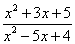(b). f(x) =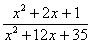Q.10. Find the domain of the following function given by 1 f(x) = x çx ê 2. f(x) =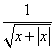3. f(x) = [x] + x Q.11. Find the range of following functions: (i). 1f(x) =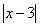(ii) 2f(x) =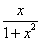(iii) nf(x) =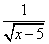(iv) f(x) = 1 - êx – 2 ç (v) f(x) =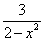(vi) f(x) = x2 + 6 (vii) f(x) =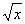(viii) f(x) =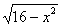Q.12. Let f =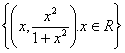be a function from R into R. Determine the range of f. Q.13. The functio n f is defined by : f(x) =Draw the graph of f(x). Q. 14. Find the domain and range of;; f(x) =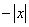. Q. 15. Draw graph of following functions: (i) f(x) =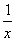(ii) f(x) = x – [x] (iii) f(x) = çx -2 ê+ êx - 3ç (iv) f(x) = { 1, if x £ 0 x2 + 1, if 0 < x < 2 (v) if; x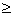;2 } 5.f(x) = { 3 – x, if x > 1 (vi) 1, if x = 1 (vii) 2x, if x < 1 } Q.16. Determine the domain and range of the relation R defined by R = {(x, x + 5) : x e ;{0, 1, 2, 3, 4, 5}}. Q.17. Let f(x) = x2 and g(x) = 2x + 1 be two real functions. Find (f – g)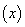,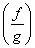. Q.18. Let f,g : R®R be defined,respectively by f(x) = x + 1,g(x) =2x – 3.Find f + g,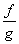. Q.19. If f(x) = [x] and g(x) =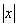;where [x] is the greatest integer function and;is the modulus function then find: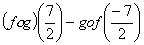. Q.20. Let f = { (1, 2); (3, 4); (-5, 6) (0, 2) } be a linear function from Z into Z. Find f(x). Q.21. Let f = {(1,1), (2,3), (0,–1), (–1, –3)} be a function from Z to Z defined by: f(x) = ax + b, for some integers a, b. Determine a, b. Paper By Mr. Anil Kumar Tondak Email Id : [email protected] Ph No.: 9811363962